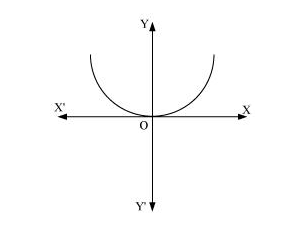# Form the differential equation of the family of parabolas having vertex at origin and axis along positive y-axis.

Question:

Form the differential equation of the family of parabolas having vertex at origin and axis along positive y-axis.

Solution:

The equation of the parabola having the vertex at origin and the axis along the positive y-axis is:

$x^{2}=4 a y$                                 ...(1)Differentiating equation (1) with respect to x, we get:

$2 x=4 a y^{\prime}$                                  ...(2)

Dividing equation (2) by equation (1), we get:

$\frac{2 x}{x^{2}}=\frac{4 a y^{\prime}}{4 a y}$

$\Rightarrow \frac{2}{x}=\frac{y^{\prime}}{y}$

$\Rightarrow x y^{\prime}=2 y$

$\Rightarrow x y^{\prime}-2 y=0$

This is the required differential equation.